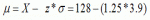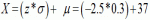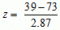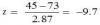# Chapter 6: Z-scores

Quizzes are available to test your understanding of the key concepts covered in each chapter. Click on the quiz below to get started.

1. What best describes a z-score:

1. It is the average of all raw scores in a normal distribution
2. It is the measure of dispersion in a distribution of scores
3. It is the position of a score relative to the mean
4. It is the frequency of a score in standardised units

The correct answer is C. a z-score is a standardised score that tells us how far the raw score is above or below the mean.

2. Which of the following statements is true about standardization?

1. It changes the units of measurement and the shape of the distribution
2. The resulting distribution has a mean of 0 and a standard deviation of 1
3. It transforms raw distributions of scores to a distribution with any desired mean and standard deviation
4. In a normal distribution, the standard deviation varies depending on the raw scores

The correct answer is B. Converting raw scores into z-scores does not change the shape of the distribution, but it sets the mean at 0 and the units of measurement are changed into standard deviation units with a standard deviation of 1.

3. Suppose that a student is told her mark in the latest test is 128 or 1.5 standard deviation units above the mean, and that the distribution of scores for the entire class is 3.9. What is the mean score of the test?

1. 125.7
2. 130
3. 123.1
4. 128.5

The correct answer is C.= 128 – 4.9 = 123.1

4. In a sample of 50 people, we have found an average corporeal temperature of 37℃, with a standard deviation of 0.3, what is the raw score if an individual has a body temperature that is 2.5 standard deviations below the mean?

1. 36.25
2. 37.75
3. 35.6
4. 36

The correct answer is A.= –0.75 + 37 = 36.25

5. The average monthly rainfall for city A is 91mm with a standard deviation of 3.58 and for a city, B is 73mm with a standard deviation of 2.87. Last month, city A registered a rainfall of 45mm and in city B 39mm. Taking last month’s scores, how much closer or further from the mean would City A’s rainfall be compared to City B if City A had the same average distribution as that of city B?

1. -21.6
2. 2.9
3. -9.75
4. -2.1

D. For City B last month’s rainfall we have:= –11.8
For City A last month’s rainfall with City B’s distribution: